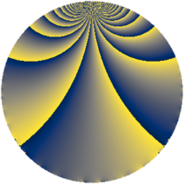# Properties

 Label 242.2.eLevel $242$ Weight $2$ Character orbit 242.e Rep. character $\chi_{242}(23,\cdot)$ Character field $\Q(\zeta_{11})$ Dimension $110$ Newform subspaces $2$ Sturm bound $66$ Trace bound $1$

# Related objects

## Defining parameters

 Level: $$N$$ $$=$$ $$242 = 2 \cdot 11^{2}$$ Weight: $$k$$ $$=$$ $$2$$ Character orbit: $$[\chi]$$ $$=$$ 242.e (of order $$11$$ and degree $$10$$) Character conductor: $$\operatorname{cond}(\chi)$$ $$=$$ $$121$$ Character field: $$\Q(\zeta_{11})$$ Newform subspaces: $$2$$ Sturm bound: $$66$$ Trace bound: $$1$$ Distinguishing $$T_p$$: $$3$$

## Dimensions

The following table gives the dimensions of various subspaces of $$M_{2}(242, [\chi])$$.

Total New Old
Modular forms 350 110 240
Cusp forms 310 110 200
Eisenstein series 40 0 40

## Trace form

 $$110q - q^{2} - 2q^{3} - 11q^{4} - 4q^{5} - 4q^{6} - 8q^{7} - q^{8} + 100q^{9} + O(q^{10})$$ $$110q - q^{2} - 2q^{3} - 11q^{4} - 4q^{5} - 4q^{6} - 8q^{7} - q^{8} + 100q^{9} + 16q^{10} + 11q^{11} + 9q^{12} + 52q^{13} + 14q^{14} + 24q^{15} - 11q^{16} - 18q^{17} - 13q^{18} - 20q^{19} - 4q^{20} - 32q^{21} + 33q^{22} - 20q^{23} - 4q^{24} - 35q^{25} - 12q^{26} - 32q^{27} - 8q^{28} - 30q^{29} - 24q^{30} + 20q^{31} - q^{32} - 44q^{33} - 14q^{34} - 48q^{35} - 21q^{36} + 38q^{37} + 48q^{38} - 56q^{39} + 16q^{40} - 42q^{41} - 28q^{42} - 44q^{43} - 11q^{44} - 68q^{45} - 24q^{46} - 40q^{47} - 2q^{48} + 29q^{49} - 31q^{50} + 49q^{51} - 14q^{52} + 18q^{53} - 40q^{54} - 22q^{55} - 8q^{56} - 69q^{57} + 20q^{58} - 54q^{59} - 20q^{60} - 62q^{61} - 32q^{62} + 6q^{63} - 11q^{64} - 18q^{65} - 33q^{66} + 60q^{67} - 18q^{68} - 80q^{69} + 4q^{70} - 16q^{71} - 13q^{72} - 30q^{73} - 38q^{74} - 102q^{75} + 35q^{76} - 44q^{77} - 48q^{78} + 30q^{79} - 4q^{80} + 14q^{81} + 14q^{82} - 84q^{83} - 32q^{84} + 112q^{85} - 34q^{86} - 120q^{87} + 22q^{88} + 80q^{89} + 98q^{90} + 110q^{91} + 68q^{92} - 34q^{93} + 128q^{94} + 56q^{95} - 4q^{96} - 4q^{97} + 31q^{98} - 33q^{99} + O(q^{100})$$

## Decomposition of $$S_{2}^{\mathrm{new}}(242, [\chi])$$ into newform subspaces

Label Dim. $$A$$ Field CM Traces $q$-expansion
$$a_2$$ $$a_3$$ $$a_5$$ $$a_7$$
242.2.e.a $$50$$ $$1.932$$ None $$5$$ $$-10$$ $$1$$ $$0$$
242.2.e.b $$60$$ $$1.932$$ None $$-6$$ $$8$$ $$-5$$ $$-8$$

## Decomposition of $$S_{2}^{\mathrm{old}}(242, [\chi])$$ into lower level spaces

$$S_{2}^{\mathrm{old}}(242, [\chi]) \cong$$ $$S_{2}^{\mathrm{new}}(121, [\chi])$$$$^{\oplus 2}$$